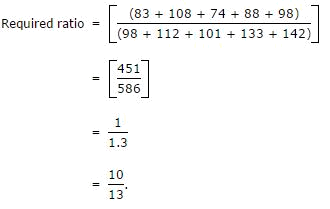# Target SBI PO Mains-  Day 12 – Aptitude – DI Table

Direction (1-4): The following table gives the percentage distribution of population of five states, P, Q, R, S and T on the basis of poverty line and also on the basis of sex.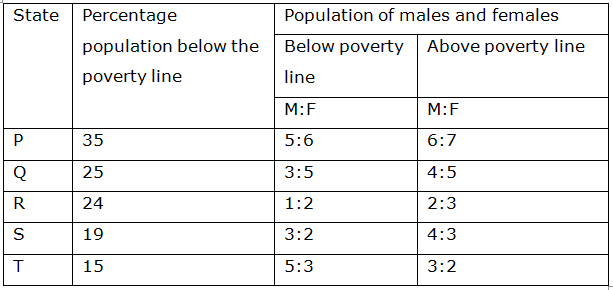1. If the male population above poverty line for State R is 1.9 million, then the total population of State R is?

(a) 4.5 million

(b) 4.85 million

(c) 5.35 million

(d) 6.25 million(e) None of these

(d) 6.25 million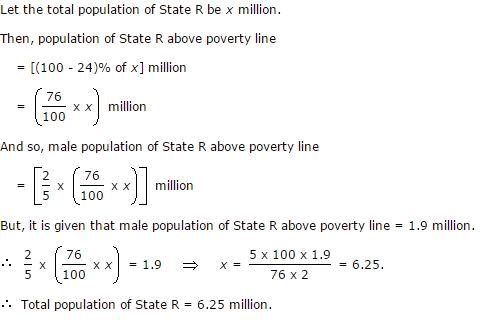2. What will be the number of females above the poverty line in the State S if it is known that the population of State S is 7 million?

(a) 3 million

(b) 2.43 million

(c) 1.33 million

(d) 5.7 million

(e) None of these

b) 2.43 million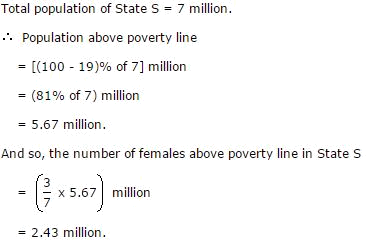3. What will be the male population above poverty line for State P if the female population below poverty line for State P is 2.1 million?

(a) 2.1 million

(b) 2.3 million

(c) 2.7 million

(d) 3.3 million

(e) None of these

(d) 3.3 million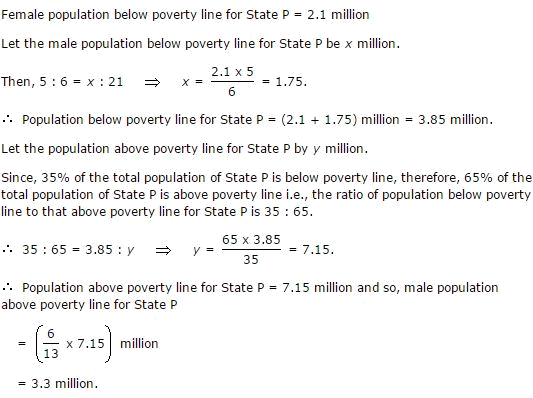4. If the population of males below poverty line for State Q is 2.4 million and that for State T is 6 million, then the total populations of States Q and T are in the ratio?

(a) 1:3

(b) 2:5

(c) 3:7

(d) 4:9

(e) None of these

(b) 2:5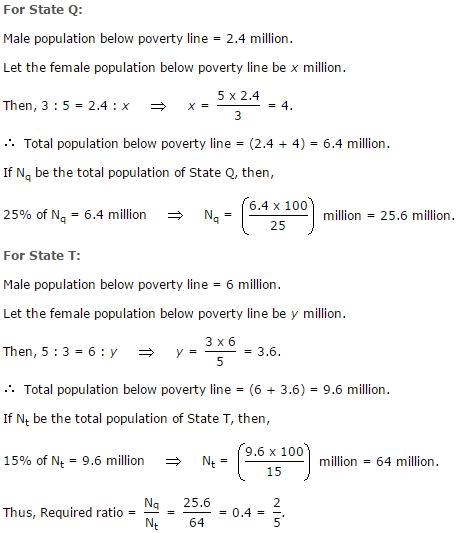Direction (5-9): Study the following table and answer the questions based on it.

Expenditures of a Company (in Lakh Rupees) per Annum Over the given Years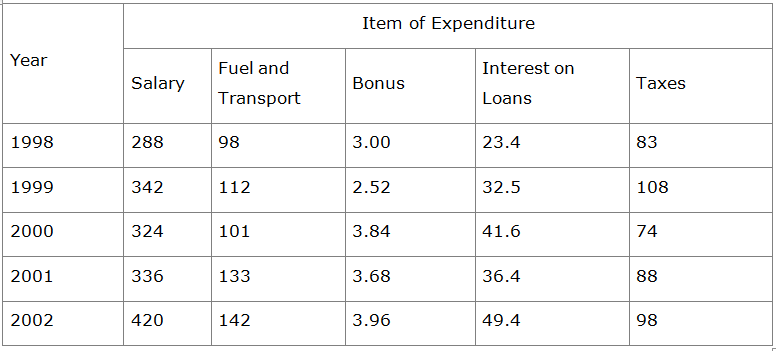5. What is the average amount of interest per year which the company had to pay during this period?

(a) 32.43 lakhs

(b) 33.72 lakhs

(c) 34.18 lakhs

(d) 36.66 lakhs

(e) None of these

(d) 36.66 lakhs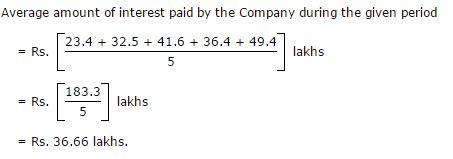6. The total amount of bonus paid by the company during the given period is approximately what percent of the total amount of salary paid during this period?

(a) 0.1%

(b) 0.5%

(c) 1%

(d) 1.25%

(e) None of these

(c) 1%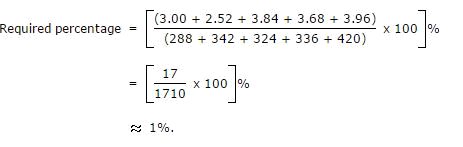7. Total expenditure on all these items in 1998 was approximately what percent of the total expenditure in 2002?

(a) 62%

(b) 66%

(c) 69%

(d) 71%

(e) None of these

(c) 69%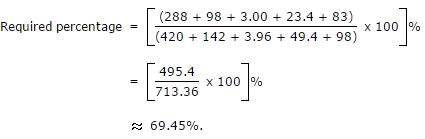8. The total expenditure of the company over these items during the year 2000 is?

(a) 544.44 lakhs

(b) 501.11 lakhs

(c) 446.46 lakhs

(d) 478.87 lakhs

(e) None of these

(a) 544.44 lakhs

Total expenditure of the Company during 2000

= Rs. (324 + 101 + 3.84 + 41.6 + 74) lakhs

= Rs. 544.44 lakhs.

9. The ratio between the total expenditure on Taxes for all the years and the total expenditure on Fuel and Transport for all the years respectively is approximately?

(a)4:7

(b) 10:13

(c) 15:18

(d) 5:8

(e) None of these